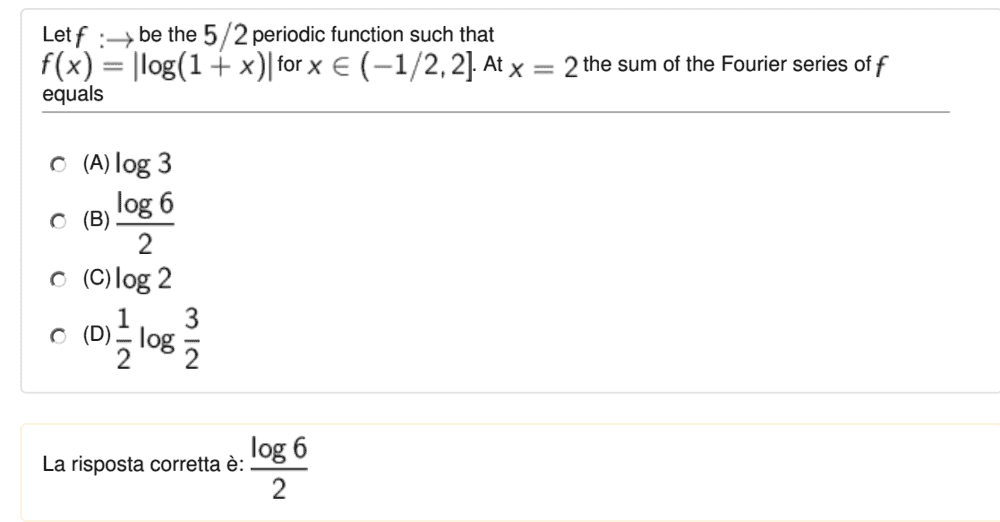# Problem with the sum of a Fourier series

• Amaelle
In summary, the Fourier sum in the region ##x=2+\epsilon\ ## bounces between ##\log 3 ## and ##\log 2 ##.f

#### Amaelle

Homework Statement
Look at the image
Relevant Equations
Fourier serie
Good dayI really don't understand how they got this result? for me the sum of the Fourier serie of of f is equal to f(2)=log(3)
any help would be highly appreciated!

Hi,
Sounds reasonable. However:
what is the sum at ##\ x=2+\epsilon\ ## with ##\ \ 0<\epsilon <<< 1\ ## ?

•Amaelle
as I saw it, I can't plug it the function f(x), so I really have no clue ...The function is periodic, so ##f(2+\epsilon) = f(-{1\over2}+\epsilon)##.
What is ## f(-{1\over2})## ?

•Amaelle
f(-1/2)=f(2)=log(3) as they are periodic, right?

And it jumps down to what when you go a little further ?

so maybe I got you
In this region the value of f(-1/2)=0 so the we are in discountinous stepwise function, in which tthe value of f(2+e)
bounces between 2 and 0?

i mean jump between log3 and 0

## f(-{1\over2}) = 0 ## ?$$\lim_{\epsilon \to 0}f(-\tfrac12 + \epsilon) = \lim_{\epsilon \to 0} |\log(-\tfrac12 + \epsilon)| = \lim_{\epsilon \to 0}\log(\tfrac{2}{1 - 2\epsilon}) = ?$$

## f(-{1\over2}) = 0 ## ?no I meant f(-1/2 +ε) =log(1+ε) =0 because -1/2 does not belong to the definition domain

$$\lim_{\epsilon \to 0}f(-\tfrac12 + \epsilon) = \lim_{\epsilon \to 0} |\log(-\tfrac12 + \epsilon)| = \lim_{\epsilon \to 0}\log(\tfrac{2}{1 - 2\epsilon}) = ?$$
log(2)

log(2)
but sorry just one question
lim f(-1/2 + epsilon)= lim |log(-1/2+epsilon)|=lim -log(-1/2+ epsilon)=lim log(2/(2epsilon-1)) I think there us a small problem with the sign no?

What is ##\bigl |\log {1\over 2} \bigr |## ?

because -1/2 does not belong to the definition domain
Correct, but does it make any difference for the Fourier series ? Do you calculate something different for ##\ (-{1\over 2}, 2]\ ## than for ##\ [-{1\over 2}, 2)\ ## ?

•Amaelle
Correct, but does it make any difference for the Fourier series ? Do you calculate something different for ##\ (-{1\over 2}, 2]\ ## than for ##\ [-{1\over 2}, 2)\ ## ?
not does not

What is ##\bigl |\log {1\over 2} \bigr |## ?
|log(2)|=log(2)

Yes; I hope you got it right: ##\ \bigl |\log{1\over 2}\bigr | = |-\log 2\bigr | = \log 2 \ .##

So at ##x=2## the Fourier sum jumps from ##\log 3 ## to ##\log 2 ##. What's the average ?

##\ ##

••hutchphd and Amaelle
log(6)/2, thanks a million!

So somehow the subtle difference between ranges ##\ (-{1\over 2}, 2]\ ## and ##\ [-{1\over 2}, 2)\ ## is 'lost in transformation'##\ ##

•Amaelle
So somehow the subtle difference between ranges ##\ (-{1\over 2}, 2]\ ## and ##\ [-{1\over 2}, 2)\ ## is 'lost in transformation'##\ ##
yes , thanks a million, that was a nice explanation!